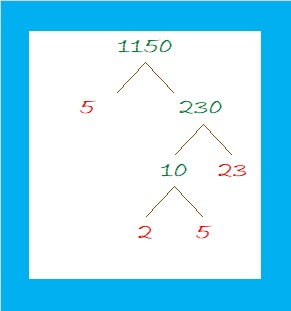# 1150 Perfectly Symmetrical Factor Trees

Factor trees can look lovely if they have symmetrical branches. 1150 can make that kind of a tree:The same number can also make a more disorderly-looking tree:All of those trees are correct factor trees. And several more can still be made for the number 1150.

What else can I tell you about that number?

• 1150 is a composite number.
• Prime factorization: 1150 = 2 × 5 × 5 × 23, which can be written 1150 = 2 × 5² × 23
• The exponents in the prime factorization are 1, 2, and 1. Adding one to each and multiplying we get (1 + 1)(2 + 1)(1 + 1) = 2 × 3 × 2 = 12. Therefore 1150 has exactly 12 factors.
• Factors of 1150: 1, 2, 5, 10, 23, 25, 46, 50, 115, 230, 575, 1150
• Factor pairs: 1150 = 1 × 1150, 2 × 575, 5 × 230, 10 × 115, 23 × 50, or 25 × 46,
• Taking the factor pair with the largest square number factor, we get √1150 = (√25)(√46) = 5√46 ≈ 33.911651150 is the hypotenuse of two Pythagorean triples:
690-920-1150 which is (3-4-5) times 230
322-1104-1150 which is (7-24-25) times 46

1150 looks interesting when it is written in a couple of different bases:
It’s 3232 in BASE 7 because 3(7³) + 2(7²) + 3(7) + 2(1) = 1150
and 6A6 in BASE 13 (A is 10 base 10) because 6(13²) + 10(13) + 6(1) = 1150

This site uses Akismet to reduce spam. Learn how your comment data is processed.